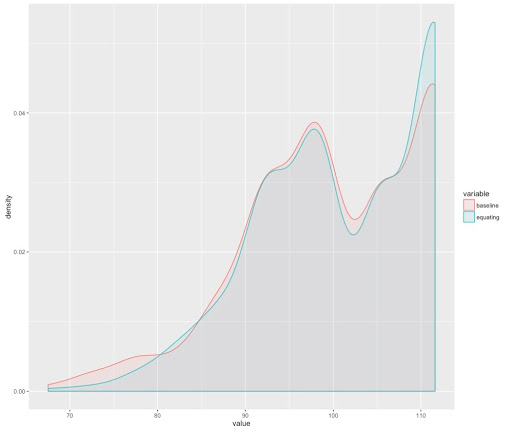Want to share your content on R-bloggers? click here if you have a blog, or here if you don't.

The main goal of standardised tests is to produce scores that can be compared not only within subgroups of students (and subpopulations of interest) but between applications (in different times). In summary, researchers and methodologists must assure that all of the scores induced by the test are in the same scale in order to allow for direct score comparisons.

If you have a baseline test, you can use the anchoring technique in order to achieve such a goal. That is, the process of estimating item parameters is carried out only for data recollected in the baseline. However, if you have a well consolidated item bank (a repository of test items) that has been validated through pilot field tests, you can even miss this step and use those very item parameters both in the baseline and in the follow-ups.Let’s suppose that a well calibrated item bank is not available. This way, you apply the test for the first time and you estimate the item parameters with the population that applied the test. Note that this process defines a scale. In the follow-up, you apply (once again) the same form to another set of individuals. However, your item parameters are fixed (to be the same that the estimation on the baseline) and you do not estimate them again. This way, your estimation of student abilities (in the follow-up) will be on the same scale that the one in the baseline. In summary, you follow the following steps:

1. You apply the test for the first time and estimate both item parameters and student abilities.
2. You apply the test for the second time.
3. With data from step 2 you: do not estimate any item parameter, but estimate the abilities of students while fixing item parameters to those values you estimate in step 1.
4. You can use any equating method (with abilities found in step 3) in order to keep the baseline scale.
5. Now you can compare scores directly and easily because the scores (at both times) are in the same scale.

The following chart may be useful for you to understand this anchoring process.So, in R you can use the following code in order to estimate the item parameters and abilities with baseline items and students, respectively. Note the inclusion of the mean/sigma method. This process finds proper constants that will be applied (always) for the rest of the applications. For this stage, the mean is 100 and the sd is 10.

```rm(list = ls())
set.seed(987654)

library(ltm)
library(mirt)
library(dplyr)
library(ggplot2)

data(LSAT)
LSAT <- sample_frac(LSAT)

N <- 500

###################
## Baseline ##
##################

LSAT.0 <- LSAT[1:N,]
fit.0 <- mirt(LSAT.0, 1, itemtype = '2PL')
coef.fit.0 <- coef(fit.0, simplify = TRUE)\$items
#coef.fit.0 <- coef(fit.0, IRTpars = TRUE, simplify = TRUE)\$items[, c(1, 2)]

# Mean/sigma
z0 <- fscores(fit.0)
b1 <- (10 / sd(z0))
b0 <- 100 - b1 * mean(z0)

#Verify that mean and sd are the same on baseline
x0 <- b0 + b1 * z0
mean(x0)
sd(x0) ```

Now, you should assure that the parameters found in the baseline remains the same for the estimation of abilities in the follow-up. I you use the mirt package, this can be done by means of the following code.

```###################
## Fixing parameters ##
##################

sv <- mirt(LSAT.0, 1, itemtype = '2PL', pars = 'values')
#custom discrimination, easiness, and guessing values
sv\$value[sv\$name == 'a1'] <- coef.fit.0[,1]
sv\$value[sv\$name == 'd'] <- coef.fit.0[,2]
#set the parameters as fixed
sv\$est <- FALSE```

Finally, you estimate abilities in the follow-up while fixing the item parameters. Note that the coefficients (item parameters) for both models [fitted in the baseline and in the follow-up] are exactly the same. Now you can compare means because the scores are in the same scale.

```########################
## Follow-up ##
########################

LSAT.1 <- LSAT[(N + 1):1000,]
fit.1 <- mirt(LSAT.1, 1, pars = sv)
coef.fit.1 <- coef(fit.1, simplify = TRUE)\$items

coef.fit.0
coef.fit.1

z1 <- fscores(fit.1)
x1 <- b0 + b1 * z1

mean(x1)
sd(x1)

# Direct comparison are now allowed

mean(x1) - mean(x0)```

Finally, for this particular case, the following plot show the densities of scores at baseline and follow-up. Note that both densities are on the same scale although the mean and sd of both forms are not the same.